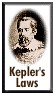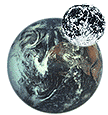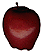# Newton's Law of GravityThe Law of Gravity was published by Sir Isaac Newton in 1687. He devised it to explain the mutual attraction experienced by all bodies of matter, such as an apple dropping to the Earth when the tree lets go of it. Newton suspected that the force pulling the apple down was the same one holding the Moon in its orbit. He went so far as to calculate the strength of this force at the distance of the Moon. In doing so, he determined that gravity falls off as one over the square of the distance to the center of the Earth.

This relationship is known as the inverse-square law of gravity, and is expressed

Force = G m1m2 /d2.
Here, m1 and m2 are the masses of the two objects, and d is the distance between them. G is called the gravitational constant.Newton showed that an object moving under the inverse-square law of gravity follows an elliptical path. Since Johannes Kepler had discovered that the planets move in ellipses, Newton concluded that they also obeyed his law of gravity. The force that made apples fall to the ground was the same that affected the planets. In a bold leap, Newton then conjectured that the law of gravity was valid throughout the universe.

The inverse-square relation also applies to light and sound. The intensity of light or sound falls off as one over the square of the distance from the source. For example, doubling your distance from a disturbing noise decreases its intensity (and presumably your annoyance) by a factor of four. A useful fact to remember.

## Gravity and the MoonUsing Newton's Law of Gravity, we can determine the force that the Earth exerts on the Moon.

In the metric system, the masses are in kilograms, the distance in meters and the value for the force is given in Newtons. The Gravitational constant G is 6.67 x 10-11 N m2 kg2 (0.0000000000667 Newton meters squared per kilogram squared). The mass of the Earth is 5.975 x 1024 kg; the mass of the Moon is 7.35 x 1022 kg; the average distance between them is 3.84 x 108 meters.Plugging these values into the equation for Newton's Law gives us a force of 1.99 x 1020 Newtons.

How does this compare to anything familiar? If an apple weighs about a third of a pound, then the force acting upon it at the surface of the Earth is 1.34 Newtons. The force needed to hold the Moon in its orbit is about 200,000,000,000,000,000,000 Newtons. Something to consider the next time you're weighing apples at the grocery store.

Back to the Story